The OpenFOAM Foundation
logPolynomialTransport< Thermo, PolySize > Class Template Reference

Transport package using polynomial functions of ln(T) for mu and kappa: More...

Inheritance diagram for logPolynomialTransport< Thermo, PolySize >: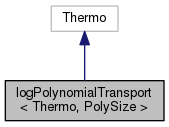[legend]
Collaboration diagram for logPolynomialTransport< Thermo, PolySize >:[legend]

## Public Member Functions

logPolynomialTransport (const logPolynomialTransport &)
Construct copy. More...

logPolynomialTransport (const word &, const logPolynomialTransport &)
Construct as named copy. More...

logPolynomialTransport (Istream &is)
Construct from Istream. More...

logPolynomialTransport (const dictionary &dict)
Construct from dictionary. More...

autoPtr< logPolynomialTransportclone () const
Construct and return a clone. More...

scalar mu (const scalar p, const scalar T) const
Dynamic viscosity [kg/ms]. More...

scalar kappa (const scalar p, const scalar T) const
Thermal conductivity [W/mK]. More...

scalar alphah (const scalar p, const scalar T) const
Thermal diffusivity of enthalpy [kg/ms]. More...

void write (Ostream &os) const
Write to Ostream. More...

void operator= (const logPolynomialTransport &)

void operator+= (const logPolynomialTransport &)

void operator-= (const logPolynomialTransport &)

void operator*= (const scalar)

## Static Public Member Functions

static autoPtr< logPolynomialTransportNew (Istream &is)

static autoPtr< logPolynomialTransportNew (const dictionary &dict)

static word typeName ()
Return the instantiated type name. More...

## Friends

logPolynomialTransport operator+ (const logPolynomialTransport &, const logPolynomialTransport &)

logPolynomialTransport operator- (const logPolynomialTransport &, const logPolynomialTransport &)

logPolynomialTransport operator* (const scalar, const logPolynomialTransport &)

logPolynomialTransport operator== (const logPolynomialTransport &, const logPolynomialTransport &)

Ostreamoperator (Ostream &, const logPolynomialTransport &)

## Detailed Description

### template<class Thermo, int PolySize = 8> class Foam::logPolynomialTransport< Thermo, PolySize >

Transport package using polynomial functions of ln(T) for mu and kappa:

ln(mu) = sum_i=1^N( a[i] * ln(T)^(i-1) ) ln(kappa) = sum_i=1^N( b[i] * ln(T)^(i-1) )

Source files

Definition at line 50 of file logPolynomialTransport.H.

## Constructor & Destructor Documentation

 logPolynomialTransport ( const logPolynomialTransport< Thermo, PolySize > & pt )
inline

Construct copy.

Definition at line 32 of file logPolynomialTransportI.H.

 logPolynomialTransport ( const word & name, const logPolynomialTransport< Thermo, PolySize > & pt )
inline

Construct as named copy.

Definition at line 58 of file logPolynomialTransportI.H.

 logPolynomialTransport ( Istream & is )

Construct from Istream.

Definition at line 33 of file logPolynomialTransport.C.

 logPolynomialTransport ( const dictionary & dict )

Construct from dictionary.

Definition at line 48 of file logPolynomialTransport.C.

## Member Function Documentation

 Foam::autoPtr< Foam::logPolynomialTransport< Thermo, PolySize > > clone ( ) const
inline

Construct and return a clone.

Definition at line 71 of file logPolynomialTransportI.H.

 Foam::autoPtr< Foam::logPolynomialTransport< Thermo, PolySize > > New ( Istream & is )
inlinestatic

Definition at line 82 of file logPolynomialTransportI.H.

 Foam::autoPtr< Foam::logPolynomialTransport< Thermo, PolySize > > New ( const dictionary & dict )
inlinestatic

Definition at line 93 of file logPolynomialTransportI.H.

References dict, and logPolynomialTransport< Thermo, PolySize >::mu().

Here is the call graph for this function: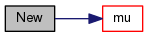static word typeName ( )
inlinestatic

Return the instantiated type name.

Definition at line 155 of file logPolynomialTransport.H.

Here is the call graph for this function: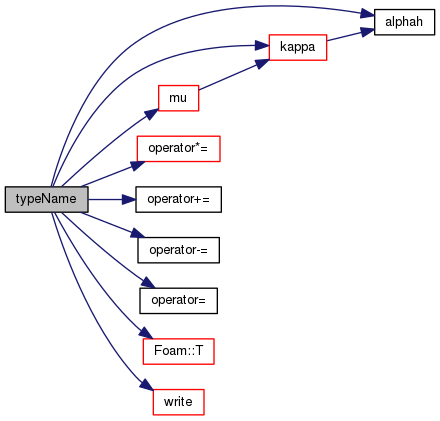Foam::scalar mu ( const scalar p, const scalar T ) const
inline

Dynamic viscosity [kg/ms].

Definition at line 106 of file logPolynomialTransportI.H.

Here is the call graph for this function: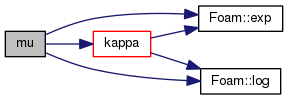Here is the caller graph for this function: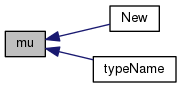Foam::scalar kappa ( const scalar p, const scalar T ) const
inline

Thermal conductivity [W/mK].

Definition at line 117 of file logPolynomialTransportI.H.

Here is the call graph for this function: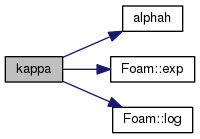Here is the caller graph for this function: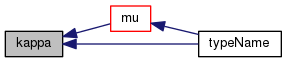Foam::scalar alphah ( const scalar p, const scalar T ) const
inline

Thermal diffusivity of enthalpy [kg/ms].

Definition at line 128 of file logPolynomialTransportI.H.

References Foam::constant::electromagnetic::kappa.

Here is the caller graph for this function: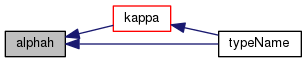void write ( Ostream & os ) const

Write to Ostream.

Definition at line 76 of file logPolynomialTransport.C.

Referenced by logPolynomialTransport< Thermo, PolySize >::typeName().

Here is the call graph for this function: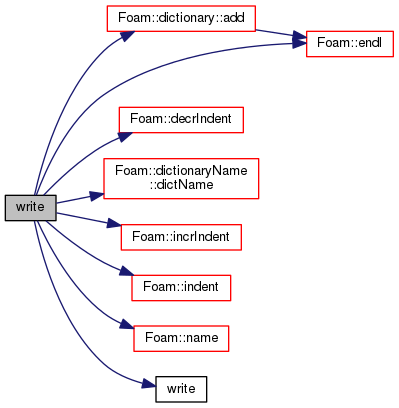Here is the caller graph for this function: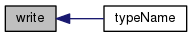void operator= ( const logPolynomialTransport< Thermo, PolySize > & )
inline

Definition at line 140 of file logPolynomialTransportI.H.

Referenced by logPolynomialTransport< Thermo, PolySize >::typeName().

Here is the caller graph for this function: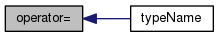void operator+= ( const logPolynomialTransport< Thermo, PolySize > & )
inline

Definition at line 153 of file logPolynomialTransportI.H.

Referenced by logPolynomialTransport< Thermo, PolySize >::typeName().

Here is the caller graph for this function: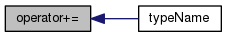void operator-= ( const logPolynomialTransport< Thermo, PolySize > & )
inline

Definition at line 171 of file logPolynomialTransportI.H.

Referenced by logPolynomialTransport< Thermo, PolySize >::typeName().

Here is the caller graph for this function: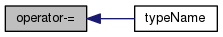void operator*= ( const scalar s )
inline

Definition at line 189 of file logPolynomialTransportI.H.

References s().

Referenced by logPolynomialTransport< Thermo, PolySize >::typeName().

Here is the call graph for this function: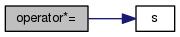Here is the caller graph for this function: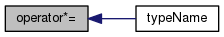## Friends And Related Function Documentation

 logPolynomialTransport operator+ ( const logPolynomialTransport< Thermo, PolySize > & , const logPolynomialTransport< Thermo, PolySize > & )
friend
 logPolynomialTransport operator- ( const logPolynomialTransport< Thermo, PolySize > & , const logPolynomialTransport< Thermo, PolySize > & )
friend
 logPolynomialTransport operator* ( const scalar , const logPolynomialTransport< Thermo, PolySize > & )
friend
 logPolynomialTransport operator== ( const logPolynomialTransport< Thermo, PolySize > & , const logPolynomialTransport< Thermo, PolySize > & )
friend
 Ostream& operator ( Ostream & , const logPolynomialTransport< Thermo, PolySize > & )
friend

The documentation for this class was generated from the following files: